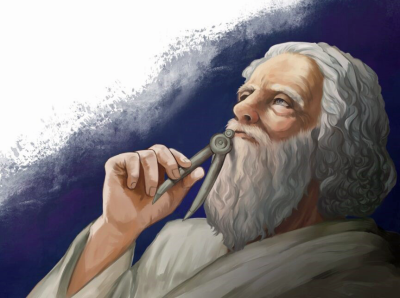# The Magic of Pi – An Introduction for KidsThe Magic of Pi – An Introduction for Kids
Archimedes was a Greek mathematician, scientist, engineer, and inventor from the town of Syracuse on the island of Sicily who lived from 287 to 212 BC. He is regarded as one of the leading scientists of classical antiquity.

As noted on Wikipedia, “Archimedes anticipated modern calculus and analysis by applying the concept of the infinitely small and the method of exhaustion to derive and rigorously prove a range of geometrical theorems, including: the area of a circle; the surface area and volume of a sphere; area of an ellipse; the area under a parabola; the volume of a segment of a paraboloid of revolution; the volume of a segment of a hyperboloid of revolution; and the area of a spiral.”

Archimedes discovered that if you divided the circumference of a circle by its diameter you would come up with the same approximate number every time. He was sure the first three digits of the number were 3.14, but wanted a more precise answer in order to derive the exact area of a circle of a given size. A nice introduction to Archimedes’ research on Pi written for younger kids is posted on Theepochtimes.com. A fascinating description of Archimedes’ work is posted on Wikipedia. Pi shows up in many other interesting contexts in mathematics, some hardly expected—watch “The Most Unusual Ways Pi Shows Up In Mathematics” to learn more! Illustrations by R.W.